# Perturbed Current Density

Let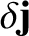be the perturbed current density associated with the tearing mode. We can write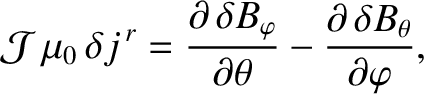(14.55)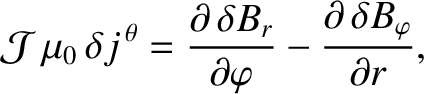(14.56)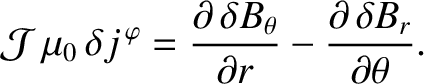(14.57)

Normally, according to our previous assumptions, all three contravariant components ofare zero. Consider, however, the behavior in the vicinity of the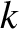th rational surface,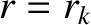, at which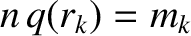. (See Section 3.7.) In general,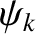,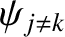, and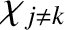are continuous across the surface, whereas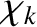is discontinuous [11,14]. Hence, we deduce that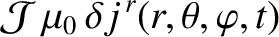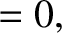(14.58)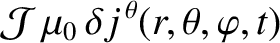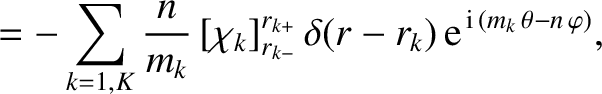(14.59)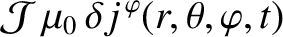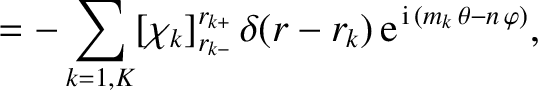(14.60)

where use has been made of Equations (14.45) and (14.54). Here, it is assumed that there are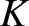rational surfaces in the plasma, numbered sequentially from 1 to, in the order of the innermost to the outermost. It is easily demonstrated from Equations (14.7)–(14.9), (14.19)–(14.22), and (14.58)–(14.60) that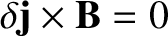at a given rational surface. Thus, we conclude that a current sheet forms at each rational surface in the plasma. Moreover, each sheet is made up of current filaments that flow parallel to the local equilibrium magnetic field.